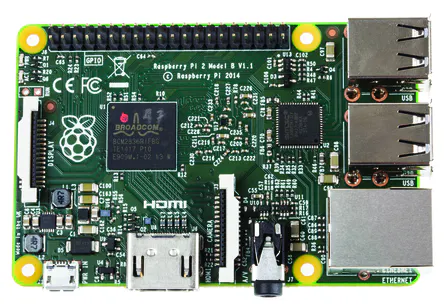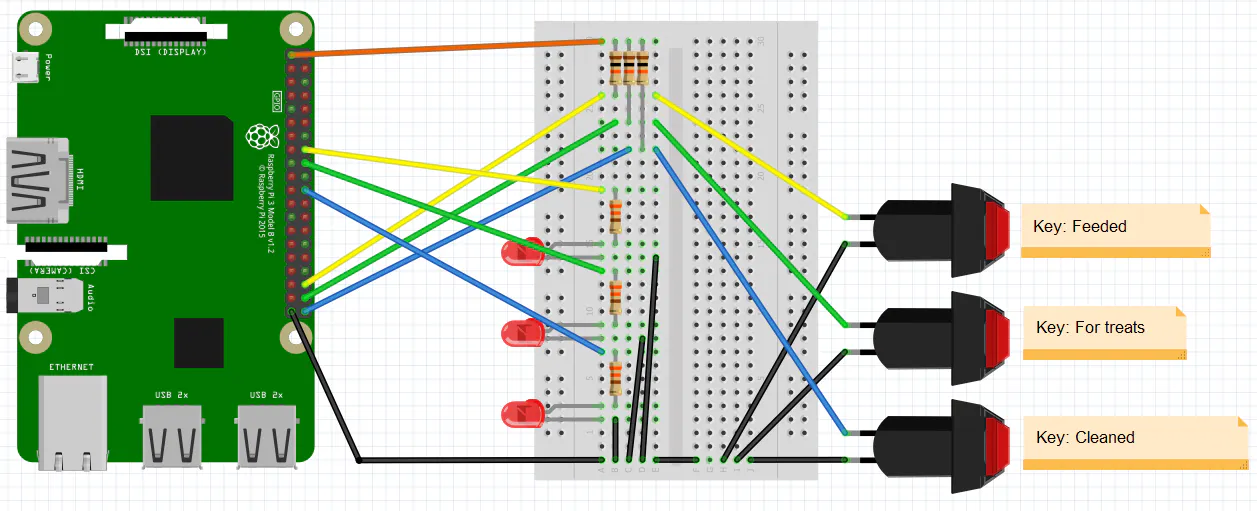# Rabbits, Buttons and Lean-Management

If someone has children and pets and several problems with both, check out my story and attempt to solve them with a Raspberry.

BeginnerShowcase (no instructions)4 hours1,306## Things used in this project

### Hardware componentsRaspberry Pi 2 Model B
×1Raspberry Pi 3 Model B
×1

## Schematics

### kaninchen_schaltung_en_iRYDgsUEnn.png## Code

### rabbits.py

Python
This is a test program for the rabbit console.
```#!/usr/bin/python
# coding=utf-8

##################################################################################################
# Rabbit console
#
# (C)2017 T. Angielsky
##################################################################################################

import time
import datetime
import RPi.GPIO as GPIO
import smtplib,sys
import os
from os.path import basename
from email.mime.application import MIMEApplication
from email.mime.image import MIMEImage
from email.mime.multipart import MIMEMultipart
from email.mime.text import MIMEText
import shutil
from decimal import *

##################################################################################################
# declaration

##################################################################################################
# common procedures

# let a LED flash: pin, length
GPIO.output(pin, True)
time.sleep(dauer)
GPIO.output(pin, False)
time.sleep(dauer)
GPIO.output(pin, True)
time.sleep(dauer)
GPIO.output(pin, False)
time.sleep(dauer)
GPIO.output(pin, True)
time.sleep(dauer)
GPIO.output(pin, False)
return

# send an email: to, subject, body
def MailVersenden(sto,betreff,txt):
try:
sfrom='Smarthome <name@deinserver.de>'
msg = MIMEText(txt,'plain','utf-8')
msg['From']=sfrom
msg['To']=sto

smtp=smtplib.SMTP('smtp.1und1.de')
smtp.starttls()
smtp.sendmail(sfrom,[sto],msg.as_string())
smtp.quit()
except:
print('Error sending mail')

# post log
def KaninchenLogEintrag(name):
tex.write(time.strftime('%d.%m.%Y %H:%M:%S')+' '+name+'\n')
tex.close()
print("Log is written.")

##################################################################################################
# Objects
# event of a keypress
class EreignisTaster:
def __init__(self,PinTaster,PinLed,Name,DateinameProtokoll,DateinameStatus):
self.PinTaster=PinTaster
self.PinLed=PinLed
self.Name=Name
self.DateinameProtokoll=DateinameProtokoll
self.DateinameStatus=DateinameStatus
self.Status=False
self.gedrueckt=0
self.lastKeypress=0
self.PruefungIntervall="-"
self.PruefungErfolgt=0
self.Meldung=""

def Pruefung(self):
if GPIO.input(self.PinTaster)==False:
if self.gedrueckt==0:
#key is pressed
self.gedrueckt=1
self.lastKeypress=datetime.datetime.now()
print("Key "+self.Name+": recognized")
else:
if (self.gedrueckt==1) and (datetime.datetime.now()-self.lastKeypress>datetime.timedelta(milliseconds=200)):
#show state
print("Key "+self.Name+": show state")
self.lastKeypress=datetime.datetime.now()
self.gedrueckt=2
#LED on
GPIO.output(self.PinLed,True)

if (self.gedrueckt==2) and (datetime.datetime.now()-self.lastKeypress>datetime.timedelta(milliseconds=1000)):
print("Key "+self.Name+": event recognized")
self.Status=True
GPIO.output(self.PinLed,True)

KaninchenLogEintrag(self.Name)

#second log, maybe for a webserver
tex.write('\n'+time.strftime('%d.%m.%Y %H:%M:%S'))
tex.close()

#write timestamp
tex.write(time.strftime('%d.%m.%Y %H:%M:%S'))
tex.close()

self.lastKeypress=datetime.datetime.now()

#show state
def StatusZeigen(self,tick):
if self.gedrueckt==2:
#show state 10 seconds
if self.Status==False:
if (tick%2==0):
GPIO.output(self.PinLed,True)
else:
GPIO.output(self.PinLed,False)

if (datetime.datetime.now()-self.lastKeypress>datetime.timedelta(milliseconds=10000)):
self.gedrueckt=0
#LED off
GPIO.output(self.PinLed,False)

##################################################################################################
#definitions

Taster=[0,1,2]
Taster=EreignisTaster(12,22,"Feeded","kaninchen_gefuettert.txt","kaninchen_gefuettert2.txt")
Taster=EreignisTaster(16, 9,"For treats","kaninchen_leckerli.txt","kaninchen_leckerli2.txt")
Taster=EreignisTaster(20,11,"Cleaned","kaninchen_saubergemacht.txt","kaninchen_saubergemacht2.txt")

Taster.PruefungIntervall="*-15:18"
Taster.Meldung="It is later than 19:00 and the rabbits weren't feeded."

#Enter number for weekday for weekly or * for daily
#0: Sunday
#1: Monday
#...
#6: Saturday

Taster.PruefungIntervall="0-12:00"
Taster.Meldung="It is Sunday and later than 12:00 and the rabbits stable wasn't cleaned."

GPIO.setwarnings(False)
GPIO.setmode(GPIO.BCM)

for t in Taster:
GPIO.setup(t.PinLed, GPIO.OUT)
GPIO.setup(t.PinTaster, GPIO.IN)
GPIO.output(t.PinLed,False)

print("Wait for signals...")
signal0h="-"
tick=0

try:
while True:
for t in Taster:
t.Pruefung()
t.StatusZeigen(tick)

time.sleep(0.2)

tick=tick+1
if tick>300: #Check every minute: are reminders necessary?
tick=0

s=time.strftime("%Y.%m.%d")
#reset the counter at 23:59
if s!=signal0h:
if time.strftime("%H:%M")=="23:59":
signal0h=s
for t in Taster:
if t.PruefungIntervall!="-":
if t.PruefungIntervall=="*":
#if daily interval, then reset
t.Status=False
t.PruefungErfolgt="-"
print("Daily reset: "+t.Name)
else:
#if weekly, then check if day fits
if time.strftime("%w")==t.PruefungIntervall:
t.Status=False
t.PruefungErfolgt="-"
print("Daily reset: "+t.Name)

#Check if reminder is necessary?
for t in Taster:
if t.PruefungIntervall!="-":
if s!=t.PruefungErfolgt:
if ((t.PruefungIntervall=="*") and (time.strftime("%H:%M")==t.PruefungIntervall[2:])) or (time.strftime("%w-%H:%M")==t.PruefungIntervall):
#it is the first time of the check
t.PruefungErfolgt="X" #because of this, there's no further check
if t.Status==False:
#MailVersenden('Thomas Angielsky <yourname@yourserver.com>','Hinweis',t.Meldung)
print(t.Meldung)

except KeyboardInterrupt:
GPIO.cleanup()
sys.exit()
```

## Credits

### Thomas Angielsky

18 projects • 31 followers
Mechanical engineer, maker, love woodwork, like Lazarus